Maths-
General
Easy

Question

# Let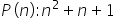is an even integer. If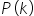is assumed true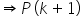is true. Therefore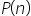is true :

## for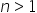for all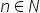for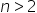for noHint:

## The correct answer is: for no### Given :is an even integerLet this be true for n = kP(k)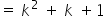is an even integerThis is also true for P(k +1)P(k +1) =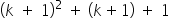P(k +1) =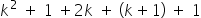P(k +1) =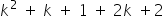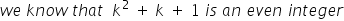#### With Turito Foundation.#### Get an Expert Advice From Turito.